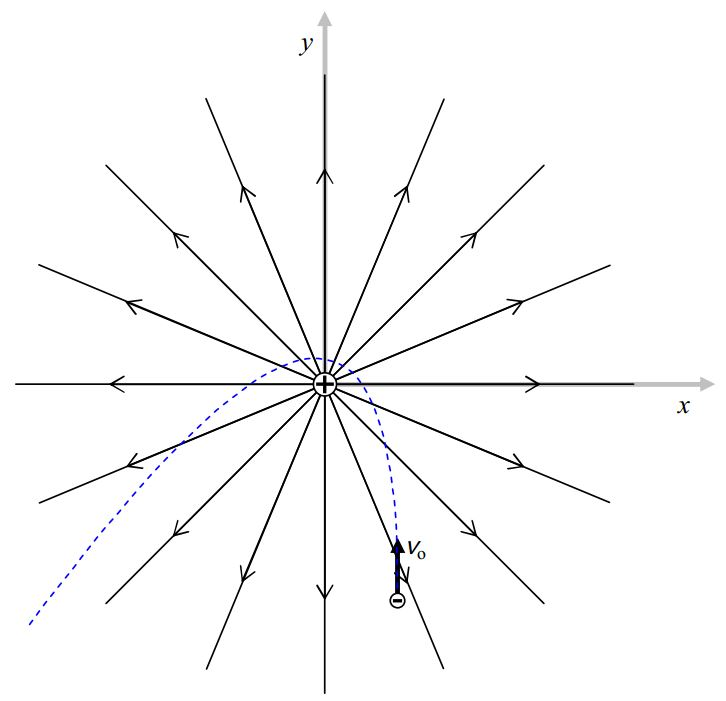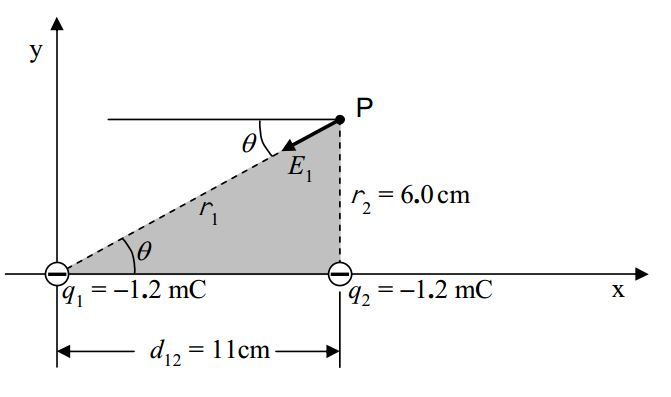# B3: The Electric Field Due to one or more Point Charges

$$\newcommand{\vecs}{\overset { \rightharpoonup} {\mathbf{#1}} }$$ $$\newcommand{\vecd}{\overset{-\!-\!\rightharpoonup}{\vphantom{a}\smash {#1}}}$$$$\newcommand{\id}{\mathrm{id}}$$ $$\newcommand{\Span}{\mathrm{span}}$$ $$\newcommand{\kernel}{\mathrm{null}\,}$$ $$\newcommand{\range}{\mathrm{range}\,}$$ $$\newcommand{\RealPart}{\mathrm{Re}}$$ $$\newcommand{\ImaginaryPart}{\mathrm{Im}}$$ $$\newcommand{\Argument}{\mathrm{Arg}}$$ $$\newcommand{\norm}{\| #1 \|}$$ $$\newcommand{\inner}{\langle #1, #2 \rangle}$$ $$\newcommand{\Span}{\mathrm{span}}$$ $$\newcommand{\id}{\mathrm{id}}$$ $$\newcommand{\Span}{\mathrm{span}}$$ $$\newcommand{\kernel}{\mathrm{null}\,}$$ $$\newcommand{\range}{\mathrm{range}\,}$$ $$\newcommand{\RealPart}{\mathrm{Re}}$$ $$\newcommand{\ImaginaryPart}{\mathrm{Im}}$$ $$\newcommand{\Argument}{\mathrm{Arg}}$$ $$\newcommand{\norm}{\| #1 \|}$$ $$\newcommand{\inner}{\langle #1, #2 \rangle}$$ $$\newcommand{\Span}{\mathrm{span}}$$$$\newcommand{\AA}{\unicode[.8,0]{x212B}}$$

A charged particle (a.k.a. a point charge, a.k.a. a source charge) causes an electric field to exist in the region of space around itself. This is Coulomb’s Law for the Electric Field in conceptual form. The region of space around a charged particle is actually the rest of the universe. In practice, the electric field at points in space that are far from the source charge is negligible because the electric field due to a point charge “dies off like one over r-squared.” In other words, the electric field due to a point charge obeys an inverse square law, which means, that the electric field due to a point charge is proportional to the reciprocal of the square of the distance that the point in space, at which we wish to know the electric field, is from the point charge that is causing the electric field to exist. In equation form, Coulomb’s Law for the magnitude of the electric field due to a point charge reads

$E=\frac{k |q|}{r^2} \label{3-1}$

where

$$E$$ is the magnitude of the electric field at a point in space,

$$k$$ is the universal Coulomb constant $$k=8.99\times 10^9 \frac{N\cdot m^2}{C^2}$$,

$$q$$ is the charge of the particle that we have been calling the point charge, and

$$r$$ is the distance that the point in space, at which we want to know $$E$$, is from the point charge that is causing $$E$$.

Again, Coulomb’s Law is referred to as an inverse square law because of the way the magnitude of the electric field depends on the distance that the point of interest is from the source charge.The number of lines drawn extending out of the positive source charge is chosen arbitrarily, but, if there was another positively charged particle, with twice the charge of the first one, in the same diagram, I would need to have twice as many lines extending out of it. That is to say that the line spacing has no absolute meaning overall, but it does have some relative meaning within a single electric field diagram. Recall the convention that the closer together the electric field lines are, the stronger the electric field. Note that in the case of a field diagram for a single source charge, the lines turn out to be closer together near the charged particle than they are farther away. It turned out this way when we created the diagram to be consistent with the fact that the electric field is always directed directly away from the source charge. The bunching of the lines close to the source charge (signifying that the electric field is strong there) is consistent with the inverse square dependence of the electric field magnitude on the distance of the point of interest from the source charge.

There are a few of important points to be made here. The first one is probably pretty obvious to you, but, just to make sure: The electric field exists between the electric field lines—its existence there is implied by the lines that are drawn—we simply can’t draw lines everywhere that the electric field does exist without completely blackening every square inch of the diagram. Thus, a charged victim that finds itself at a position in between the lines will experience a force as depicted below for each of two different positively-charged victims.The next point is a reminder that a negatively-charged particle that finds itself at a position at which an electric field exists, experiences a force in the direction exactly opposite that of the electric field at that position.The third and final point that should be made here is a reminder that the direction of the force experienced by a particle, is not, in general, the direction in which the particle moves. To be sure, the expression “in general” implies that there are special circumstances in which the particle would move in the same direction as that of the electric field but these are indeed special. For a particle on which the force of the electric field is the only force acting, there is no way it will stay on one and the same electric field line (drawn or implied) unless that electric field line is straight (as in the case of the electric field due to a single particle). Even in the case of straight field lines, the only way a particle will stay on one and the same electric field line is if the particle’s initial velocity is zero, or if the particle’s initial velocity is in the exact same direction as that of the straight electric field line. The following diagram depicts a positively-charged particle, with an initial velocity directed in the $$+y$$ direction. The dashed line depicts the trajectory for the particle (for one set of initial velocity, charge, and mass values). The source charge at the origin is fixed in position by forces not specified.Here is an example of a trajectory of a negatively-charged particle, again for one set of values of source charge, victim charge, victim mass, and victim initial velocity:Again, the point here is that, in general, charged particles do not move along the electric field lines, rather, they experience a force along (or, in the case of negative particles, in the exact opposite direction to) the electric field lines.

At this point, you should know enough about electric field diagrams to construct the electric field diagram due to a single negatively-charged particle. Please do so and then compare your work with the following diagram:## Some General Statements that can be made about Electric Field Lines

The following useful facts about electric field lines can be deduced from the definitions you have already been provided:

1. Every electric field line begins either at infinity or at a positive source charge.
2. Every electric field line ends either at infinity or at a negative source charge.
3. Electric field lines never cross each other or themselves.

## Superposition

If there is more than one source charge, each source charge contributes to the electric field at every point in the vicinity of the source charges. The electric field at a point in space in the vicinity of the source charges is the vector sum of the electric field at that point due to each source charge. For instance, suppose the set of source charges consists of two charged particles. The electric field at some point $$P$$ will be the electric field vector at point $$P$$ due to the first charged particle plus the electric field vector at point $$P$$ due to the second particle. The determination of the total electric field at point $$P$$ is a vector addition problem because the two electric field vectors contributing to it are, as the name implies, vectors.

Suppose, for instance, that you were asked to find the magnitude and direction of the electric field vector at point $$P$$ due to the two charges depicted in the diagram below:given that charge $$q_1$$ is at $$(0,0)$$, $$q_2$$ is at $$(11\mbox{cm}, 0)$$ and point $$P$$ is at $$(11\mbox{cm}, 6.0\mbox{cm})$$. The first thing that you would have to do is to find the direction and magnitude of $$\vec{E}_1$$ (the electric field vector due to $$q_1$$) and the direction and magnitude of $$\vec{E}_2$$ (the electric field vector due to $$q_2$$).Referring to the diagram above, the direction of $$\vec{E}_2$$ is “the $$–y$$ direction” by inspection.

The angle $$\theta$$ specifying the direction of $$\vec{E}_1$$ can be determined by analyzing the shaded triangle in the following diagram.Analysis of the shaded triangle will also give the distance $$r_1$$ that point $$P$$ is from charge $$q_1$$. The value of $$r_1$$ can then be substituted into

$E_1=\frac{k|q_1|}{r^1_2} \nonumber$

to get the magnitude of $$\vec{E}_1$$. Based on the given coordinates, the value of $$r_2$$ is apparent by inspection and we can use it in

$E_2=\frac{k|q_2|}{r^2_2} \nonumber$

to get the magnitude of $$\vec{E}_2$$. With the magnitude and direction for both $$\vec{E}_1$$ and $$\vec{E}_2$$, you follow the vector addition recipe to arrive at your answer:

##### For each vector: a. Draw a vector component diagram. b. Analyze the vector component diagram to get the components of the vector. Add the x components to get the x component of the resultant. Add the y components to get the y component of the resultant. For the resultant: a. Draw a vector component diagram. b. Analyze the vector component diagram to get the magnitude and direction of the resultant.

This page titled B3: The Electric Field Due to one or more Point Charges is shared under a CC BY-SA 2.5 license and was authored, remixed, and/or curated by Jeffrey W. Schnick via source content that was edited to the style and standards of the LibreTexts platform; a detailed edit history is available upon request.